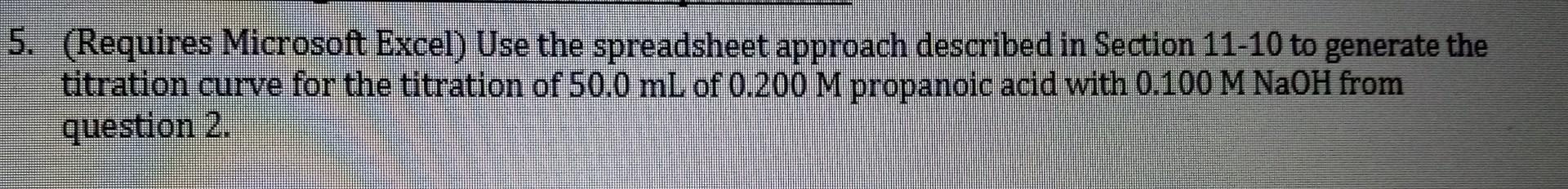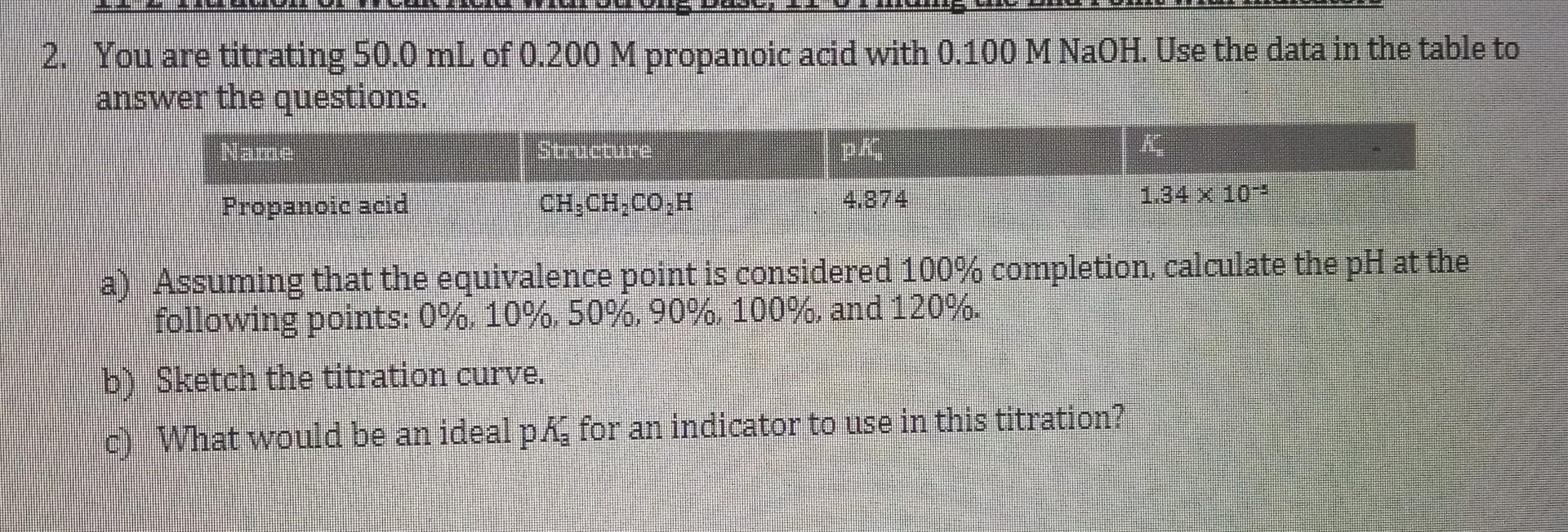# Question     (Requires Microsoft Excel) Use the spreadsheet approach described in Section 11-10 to generate the titration curve for the titration of $$50.0 \mathrm{~mL}$$ of $$0.200 \mathrm{M}$$ propanoic acid with $$0.100 \mathrm{M} \mathrm{NaOH}$$ from question 2. 2. You are titrating $$50.0 \mathrm{~mL}$$ of $$0.200 \mathrm{M}$$ propanoic acid with $$0.100 \mathrm{M} \mathrm{NaOH}$$. Use the data in the table to answer the questions. a) Assuming that the equivalence point is considered $$100 \%$$ completion, calculate the $$\mathrm{pH}$$ at the following points: $$0 \%, 10 \%, 50 \%, 90 \%, 100 \%$$, and $$120 \%$$. b) Sketch the titration curve. c) What would be an ideal $$\mathrm{p} K_{\mathrm{a}}$$ for an indicator to use in this titration?Transcribed Image Text: (Requires Microsoft Excel) Use the spreadsheet approach described in Section 11-10 to generate the titration curve for the titration of $$50.0 \mathrm{~mL}$$ of $$0.200 \mathrm{M}$$ propanoic acid with $$0.100 \mathrm{M} \mathrm{NaOH}$$ from question 2. 2. You are titrating $$50.0 \mathrm{~mL}$$ of $$0.200 \mathrm{M}$$ propanoic acid with $$0.100 \mathrm{M} \mathrm{NaOH}$$. Use the data in the table to answer the questions. a) Assuming that the equivalence point is considered $$100 \%$$ completion, calculate the $$\mathrm{pH}$$ at the following points: $$0 \%, 10 \%, 50 \%, 90 \%, 100 \%$$, and $$120 \%$$. b) Sketch the titration curve. c) What would be an ideal $$\mathrm{p} K_{\mathrm{a}}$$ for an indicator to use in this titration?
More
Transcribed Image Text: (Requires Microsoft Excel) Use the spreadsheet approach described in Section 11-10 to generate the titration curve for the titration of $$50.0 \mathrm{~mL}$$ of $$0.200 \mathrm{M}$$ propanoic acid with $$0.100 \mathrm{M} \mathrm{NaOH}$$ from question 2. 2. You are titrating $$50.0 \mathrm{~mL}$$ of $$0.200 \mathrm{M}$$ propanoic acid with $$0.100 \mathrm{M} \mathrm{NaOH}$$. Use the data in the table to answer the questions. a) Assuming that the equivalence point is considered $$100 \%$$ completion, calculate the $$\mathrm{pH}$$ at the following points: $$0 \%, 10 \%, 50 \%, 90 \%, 100 \%$$, and $$120 \%$$. b) Sketch the titration curve. c) What would be an ideal $$\mathrm{p} K_{\mathrm{a}}$$ for an indicator to use in this titration?